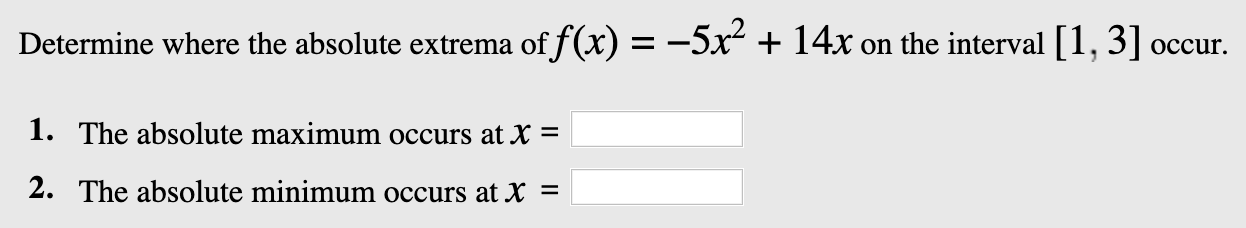# Determine where the absolute extrema of f(x) =--5x + 14x on the interval 1, 3] occur.1. The absolute maximum occurs at X =2. The absolute minimum occurs at X =

Question
9 viewshelp_outlineImage TranscriptioncloseDetermine where the absolute extrema of f(x) =--5x + 14x on the interval 1, 3] occur. 1. The absolute maximum occurs at X = 2. The absolute minimum occurs at X = fullscreen
check_circle

Step 1

Given function is

Step 2

Firstly, we find stationary points of f(x) by solving f'(x)=0

Step 3

Now, we find f(1), f(7/5...

### Want to see the full answer?

See Solution

#### Want to see this answer and more?

Solutions are written by subject experts who are available 24/7. Questions are typically answered within 1 hour.*

See Solution
*Response times may vary by subject and question.
Tagged in
MathCalculus

### Derivative